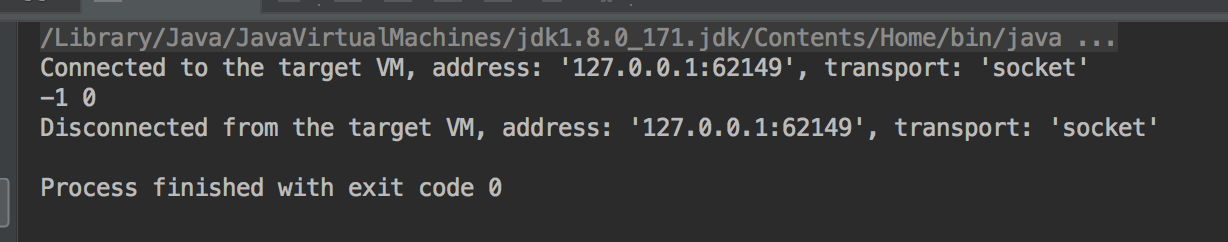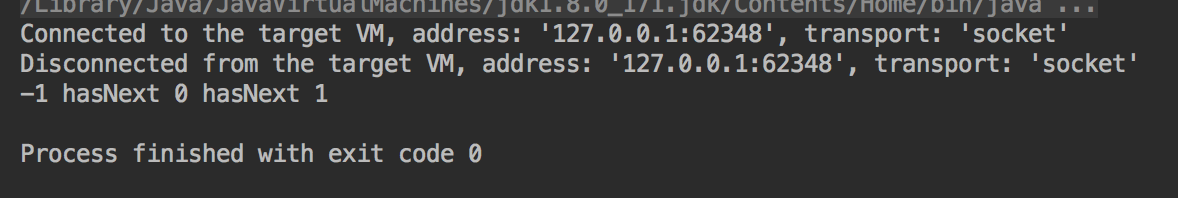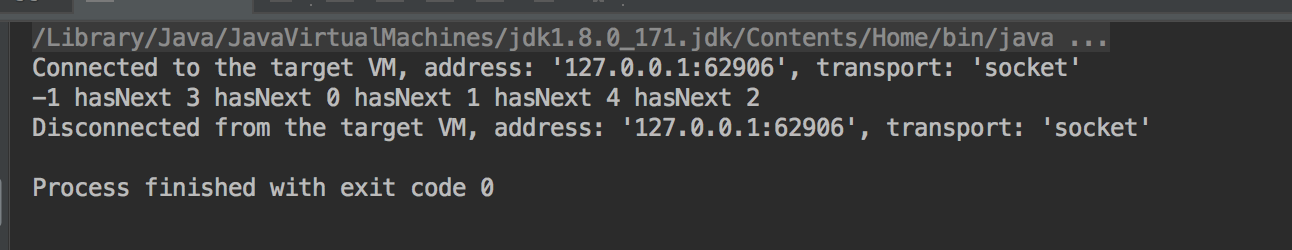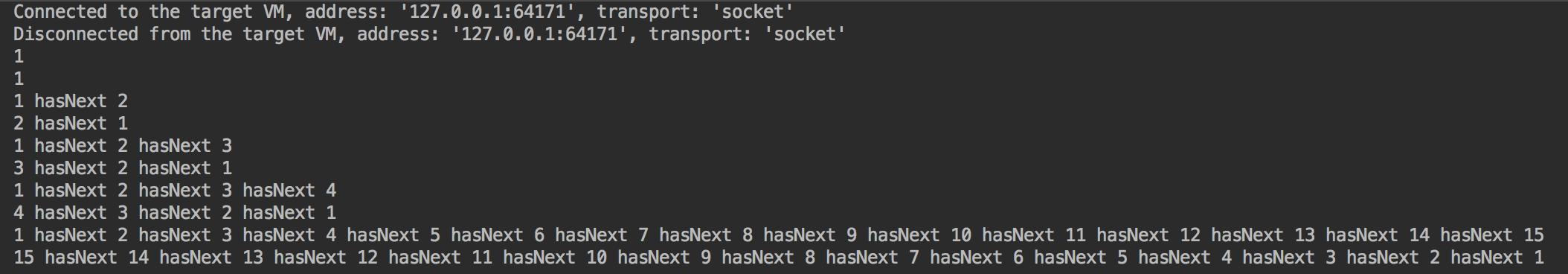# Java实现简单的链表-面向初学者

• 节点
先确定节点数据结构（一个节点一个数字好了），后续慢慢一点点扩展：
/**
* @author hsf
* @description
* @create 2018-07-14 下午3:47
**/

public class Node {
//数据
public int value;
//下一节点
public Node next;

}

/**
* @author hsf
* @description
* @create 2018-07-14 下午3:47
**/

public class Node {
//数据
private int value;
//下一节点
private Node next;

public int getValue() {
return value;
}

public Node setValue(int value) {
this.value = value;
return this;
}

public Node getNext() {
return next;
}

public Node setNext(Node next) {
this.next = next;
return this;
}
}

public static void main(String [] args){
//头节点
//第一个节点
Node firstNode = new Node();
firstNode.setValue(0);
firstNode.setNext(null);
//链接
}public Node(int value,Node next){
this.value =value;
this.next = next;
}

public Node(int value){
this.value = value;
}

public Node(){}

public boolean hasNext(){
return this.next == null ? false : true;
}

this.next = node;
return node;
}


public static void main(String [] args){
//头节点
//第一个节点
Node firstNode = new Node(0);
//第二个节点
Node secondNode = new Node(1);
//链接
}

//直接重写toString好了
@Override
public String toString() {
String val = Integer.toString(value);
if(next != null){
val = val.concat(" hasNext ");
val = val.concat(next.toString());
}
return val;
}

System.out.println(head);//先找到尾巴，在插入就好了  需要的话可以返回尾节点。
Node temp = this;
while(temp.hasNext()){
temp = temp.next;
}
temp.next = node;
}

//现将新节点的值指向原有头节点的下一个节点，再将头节点指向新节点
}

public Node addToNext(Node node){
node.next = this.next;
this.next = node;
return node;
}

public static void main(String [] args){
//头节点
//第一个节点
Node firstNode = new Node(0);
//第二个节点
Node secondNode = new Node(1);
//链接
//加入第二个节点
//向尾部添加节点
//向头部添加节点
//向中间添加节点
//输出
}• 单向链表逆转
假设只知道一个头节点。难度其实在于单向链表在没有指向前趋节点的情况下怎么获取到前驱节点，并且逆转之后怎么获取正确的原有下一节点。
先创建一个测试的方法入口并且定义好测试用的链表。

public static void main(String[] args) {
//1个
//2个
//3个
//4个
//多个
for (int i = 2; i <= 15; i++) {
}
}

• 直接在原基础链表上反转
操作：遍历原有链表，直接将其下一个节点引用反转
public static Node reverse(Node head) {
Node curNode = null;
Node afterNode = null;
return null;
}
} else {
}
if (curNode.hasNext()) {
afterNode = curNode.getNext();
} else {
return curNode;
}
//假如有3个及3个以上
//先将第一个节点独立，如果不独立则这个节点将永远指向原链表中的第二个节点，则会成环。必须切断引用。
while (afterNode.hasNext()) {
curNode = afterNode;
afterNode = afterNode.getNext();
}
afterNode.setNext(curNode);
return afterNode;
}

执行结果：public static Node reverse(Node head){
Node cur = null; //当前节点
}else{
}
pre.setNext(null); //依旧先切前驱节点的与原链表的联系
while(cur.hasNext()){
Node after = cur.getNext(); //标记出来下一个操作的节点
cur.setNext(pre); //当前节点指向前驱节点
pre = cur; //现有当前节点改为前驱节点
cur = after; //现有下一个节点 改为当前需要操作的节点
}
cur.setNext(pre); //到最末尾的时候直接反转，cur为新的头节点
return cur;
}

• 借助新链表（逻辑上的）逆转
实现逻辑：
（1） 创建一个新的链表
（2）遍历原有连标到最末端
（3）将末端节点作为新的链表的第一个节点，并且将其从原有链表上切掉（切断其与前前趋节点的连接）
之后重复（2）（3）操作，直至原链表节点全部被移动到新链表中

• 借助栈实现
这个是我第一个想到的解决方案，栈是先进后出的数据结构，则反转其实就是直接遍历并放入一个栈中，逆转则吧元素从栈中逐个取出，并重新建立一个链接关系即可。
因为需要栈支持，所以实现的前提是要有一个现成的栈，当然也可以是一个数组或者别的数据结构，因为这个逆转核心的问题是怎么解决怎么从当前节点寻找前驱节点，所以其实只要有另外一个可以支持这种查找的就可以借助来实现逆转。

• 递归实现
想法其实类似栈，有一种翻衣袖的感觉。

public static Node reverse(Node head){
//空链表 或者 已经到了尾节点 不判断尾节点会空指针。
}else{
//取出下一个节点
//先作为下一次调用的参数传到下一个节点，切记不能先处理再传
Node retNode = reverse(nextNode);
}